##### Algorithmic Graph Theory

Finally, there are several invariants of graphs which are of particular interest because of the algorithms that compute them.

The shortestŁpath spanning tree of a grid graph is defined in terms of Manhattan distance, where the distance between points with coordinates $\left(x, y\right)$ and $\left(u, v\right)$ is $|x - u| + |y - v|$.

`ShowGraph[  ShortestPathSpanningTree[    GridGraph[5,5],1] ];`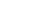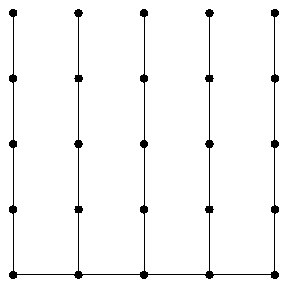In an unweighted graph there can be many different shortest paths between any pair of vertices. This path between two opposing corners goes all the way to the right, then all the way to the top.

`ShortestPath[GridGraph[5,5],1,25]`
``

A minimum spanning tree of a weighted graph is a set of $n - 1$ edges of minimum total weight which form a spanning tree of the graph. Any spanning tree is a minimum spanning tree when the graphs are unweighted.

`ShowGraph[ MinimumSpanningTree[ CompleteGraph[6,6,6] ] ];`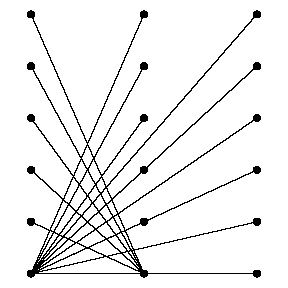The number of spanning trees of a complete graph is $n^\left\{n - 2\right\}$, as was proved by Cayley.

`NumberOfSpanningTrees[CompleteGraph]`
`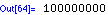`

The maximum flow through an unweighted complete bipartite graph $G$ is the minimum degree $\delta \left(G\right)$.

`NetworkFlow[ CompleteGraph[4,4], 1, 8]`
`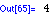`

A matching in a graph $G$ is a set of edges of $G$ such that no two of them share a vertex in common. A perfect matching of an even cycle consists of alternating edges in the cycle.

`BipartiteMatching[ Cycle ]`
`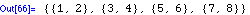`

Any maximal matching of a $K_n$ is a maximum matching, and perfect if $n$ is even.

`MaximalMatching[CompleteGraph]`
`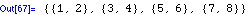`

Planar graphs are graphs which can be embedded in the plane with no pair of edges crossing. $K_\left\{3,3\right\}$ and $K_5$ are the basic nonplanar graphs.

`PlanarQ[CompleteGraph] || PlanarQ[CompleteGraph[3,3]]`
`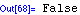`

Every planar graph on nine vertices has a nonplanar complement.

`PlanarQ[ GraphComplement[GridGraph[3,3]] ]`
`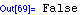`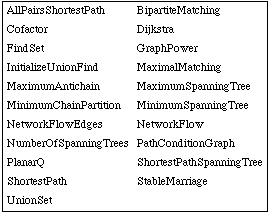Combinatorica functions for algorithmic graph theory.